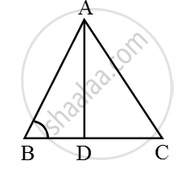Share

# In figure, ∠B of ∆ABC is an acute angle and AD ⊥ BC, prove that AC^2 = AB^2 + BC^2 – 2BC × BD - CBSE Class 10 - Mathematics

#### Question

In figure, ∠B of ∆ABC is an acute angle and AD ⊥ BC, prove that AC2 = AB2 + BC2 – 2BC × BD

#### Solution

Given: A ∆ABC in which ∠B is an acute angle and AD ⊥ BC.

To Prove: AC2 = AB2 + BC2 – 2BC × BD.

Proof: Since ∆ADB is a right triangle right-angled at D. So, by Pythagoras theorem, we have

AB2 = AD2 + BD2 ….(i)

Again ∆ADC is a right triangle right angled at D.So, by Pythagoras theorem, we have

⇒ AC2 = AD2 + (BC – BD)2

⇒ AC2 = AD2 + (BC2 + BD2 – 2BC • BD)

⇒ AC2 = (AD2 + BD2 ) + BC2 – 2BC • BD

⇒ AC2 = AB2 + BC2 – 2BC • BD [Using (i)]

Hence, AC2 = AB2 + BC2 – 2BC • BD

Is there an error in this question or solution?

#### Video TutorialsVIEW ALL 

Solution In figure, ∠B of ∆ABC is an acute angle and AD ⊥ BC, prove that AC^2 = AB^2 + BC^2 – 2BC × BD Concept: Pythagoras Theorem.
S